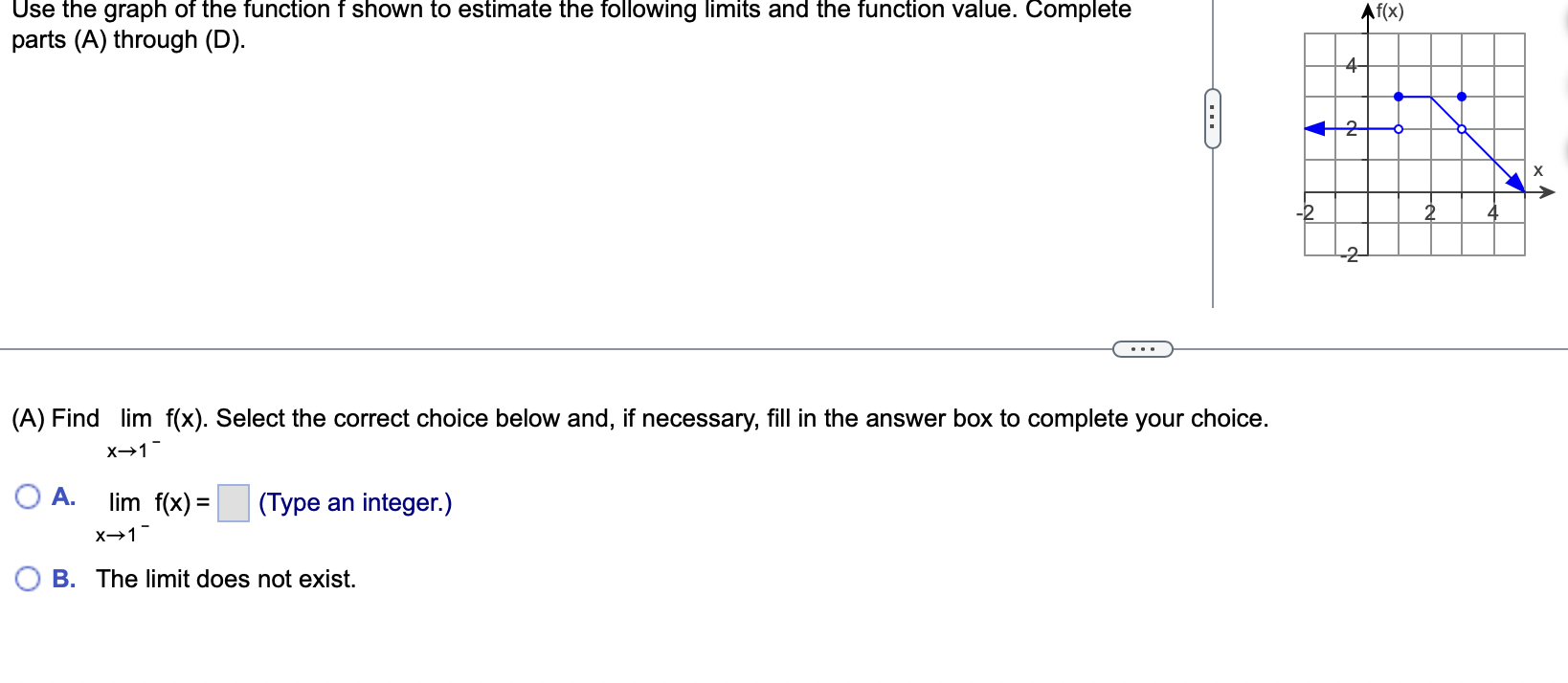Home / Expert Answers / Calculus / use-the-graph-of-the-function-f-shown-to-estimate-the-following-limits-and-the-function-valu-pa237

# (Solved): Use the graph of the function $$f$$ shown to estimate the following limits and the function valu ...Use the graph of the function $$f$$ shown to estimate the following limits and the function value. Complete parts (A) through (D). (A) Find $$\lim _{x \rightarrow 1^{-}} f(x)$$. Select the correct choice below and, if necessary, fill in the answer box to complete your choice. A. $$\lim _{x \rightarrow 1^{-}} f(x)=$$ (Type an integer.) B. The limit does not exist.

We have an Answer from Expert